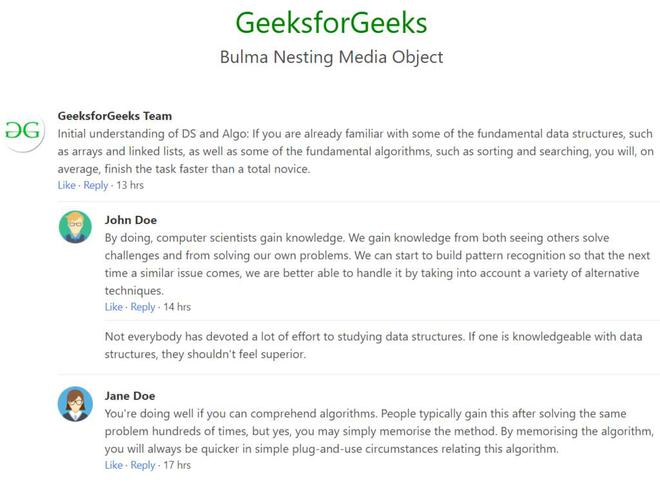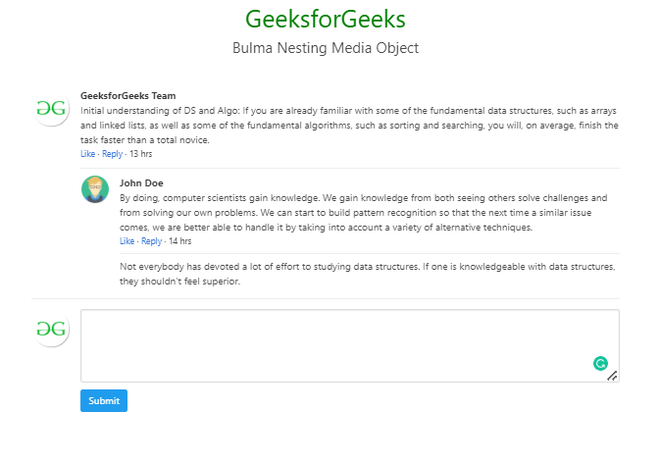# Bulma Nesting Media Object

• Last Updated : 28 Jul, 2022

Bulma is a free, open-source CSS framework based on Flexbox. It is component rich, compatible, and well documented. It is highly responsive in nature. It uses classes to implement its design.

Bulma Media Object is a component that is very relevant to use in a social media interface as a part of the common commenting section. But this is extremely useful also in e-commerce as well as blog websites and websites GeeksforGeeks itself. Nesting is also an integral part of using the media object as the commenting section because nesting helps us add and show the UI part of replying to a comment.

Bulma Nesting Media Object Classes:

• media: This class is added to the media object container.
• media-left: This class is used to add an image or icon or text to the left of the content of the media object.
• media-content: This class is added to the content container to the media content.
• content: This class is used to specify the content of the media object.
• field: This class is used to add a field/textarea to the media object.

Syntax:

```<article class="media">
<figure class="media-left">
<p>
...
</p>
</figure>
<div class="media-content">
<div class="content">
<p>
...
</p>
</div>
</div>
</article>```

Example 1: The code below demonstrates the nesting of media objects using the above-given classes.

## HTML

 ` ` `<``html``> ` ` `  `<``head``> ` `    ``<``link` `rel``=``"stylesheet"` `href``= ` `"https://cdnjs.cloudflare.com/ajax/libs/bulma/0.7.5/css/bulma.css"``> ` ` `  `    ``` `    ``<``style``> ` `        ``div.adjust { ` `            ``margin-top: 50px; ` `        ``} ` `    `` ` ` ` ` `  `<``body``> ` `    ``<``center``> ` `        ``<``h1` `style``=``"color: green; font-size: 2.5rem;"``> ` `            ``GeeksforGeeks ` `        `` ` `        ``<``h3` `style``=``"font-size: 1.5rem;"``> ` `            ``Bulma Nesting Media Object ` `        `` ` `    `` ` ` `  `    ``<``div` `class``=``'container adjust'``> ` `        ``<``article` `class``=``"media"``> ` `            ``<``figure` `class``=``"media-left"``> ` `                ``<``p` `class``=``"image is-64x64"``> ` `                    ``<``img` `src``= ` `"https://media.geeksforgeeks.org/wp-content/uploads/20200819145636/icon.png"``> ` `                `` ` ` `  `            `` ` `            ``<``div` `class``=``"media-content"``> ` `                ``<``div` `class``=``"content"``> ` `                    ``<``p``> ` `                        ``<``strong``>GeeksforGeeks Team ` `                        ``<``br``> ` `                        ``Initial understanding of DS and Algo:  ` `                        ``If you are already familiar with some  ` `                        ``of the fundamental data structures,  ` `                        ``such as arrays and linked lists, as ` `                        ``well as some of the fundamental algorithms, ` `                        ``such as sorting and searching, you will,  ` `                        ``on average, finish the task faster than  ` `                        ``a total novice. ` `                        ``<``br``> ` `                        ``<``small``><``a``>Like · <``a``>Reply  ` `                            ``· 13 hrs ` `                    `` ` `                `` ` `                ``<``article` `class``=``"media"``> ` `                    ``<``figure` `class``=``"media-left"``> ` `                        ``<``p` `class``=``"image is-48x48"``> ` `                            ``<``img` `src``= ` `"https://media.geeksforgeeks.org/wp-content/uploads/20220629143017/avatar.png"``> ` `                        `` ` `                    `` ` `                    ``<``div` `class``=``"media-content"``> ` `                        ``<``div` `class``=``"content"``> ` `                            ``<``p``> ` `                                ``<``strong``>John Doe ` `                                ``<``br``> ` `                                ``By doing, computer scientists gain  ` `                                ``knowledge. We gain knowledge from  ` `                                ``both seeing others solve challenges  ` `                                ``and from solving our own problems.  ` `                                ``We can start to build pattern  ` `                                ``recognition so that the next time a  ` `                                ``similar issue comes, we are better  ` `                                ``able to handle it by taking into  ` `                                ``account a variety of alternative  ` `                                ``techniques. ` `                                ``<``br``> ` `                                ``<``small``><``a``>Like · <``a``>Reply  ` `                                    ``· 14 hrs ` `                            `` ` ` `  `                        `` ` `                        ``<``article` `class``=``"media"``> ` `                            ``Not everybody has devoted a lot of  ` `                            ``effort to studying data structures.  ` `                            ``If one is knowledgeable with data  ` `                            ``structures, they shouldn't feel superior. ` `                        `` ` `                    `` ` `                `` ` `                ``<``article` `class``=``"media"``> ` `                    ``<``figure` `class``=``"media-left"``> ` `                        ``<``p` `class``=``"image is-48x48"``> ` `                            ``<``img` `src``= ` `"https://media.geeksforgeeks.org/wp-content/uploads/20220629143016/avatar1.png"``> ` `                        `` ` ` `  `                    `` ` `                    ``<``div` `class``=``"media-content"``> ` `                        ``<``div` `class``=``"content"``> ` ` `  `                            ``<``p``> ` `                                ``<``strong``>Jane Doe ` `                                ``<``br``> ` `                                ``You're doing well if you can comprehend  ` `                                ``algorithms. People typically gain this  ` `                                ``after solving the same problem hundreds  ` `                                ``of times, but yes, you may simply memorise  ` `                                ``the method. By memorising the algorithm, you ` `                                ``will always be quicker in simple plug-and-use ` `                                ``circumstances relating this algorithm. ` `                                ``<``br``> ` `                                ``<``small``><``a``>Like · <``a``>Reply ` `                                    ``· 17 hrs ` `                            `` ` `                        `` ` `                    `` ` `                `` ` `            `` ` `        `` ` `    `` ` ` ` ` `  ` `

Output:Example 2: The code below demonstrates the nesting of media objects using the above-given classes and adding an empty textarea for adding another comment.

## HTML

 ` ` `<``html``> ` ` `  `<``head``> ` `    ``<``link` `rel``=``"stylesheet"` `href``= ` `"https://cdnjs.cloudflare.com/ajax/libs/bulma/0.7.5/css/bulma.css"``> ` ` `  `    ``` `    ``<``style``> ` `        ``div.adjust { ` `            ``margin-top: 50px; ` `        ``} ` `    `` ` ` ` ` `  `<``body``> ` `    ``<``center``> ` `        ``<``h1` `style``=``"color: green; font-size: 2.5rem;"``> ` `            ``GeeksforGeeks ` `        `` ` `        ``<``h3` `style``=``"font-size: 1.5rem;"``> ` `            ``Bulma Nesting Media Object ` `        `` ` `    `` ` `    ``<``div` `class``=``'container adjust'``> ` `        ``<``article` `class``=``"media"``> ` `            ``<``figure` `class``=``"media-left"``> ` `                ``<``p` `class``=``"image is-64x64"``> ` `                    ``<``img` `src``= ` `"https://media.geeksforgeeks.org/wp-content/uploads/20200819145636/icon.png"``> ` `                `` ` `            `` ` `            ``<``div` `class``=``"media-content"``> ` `                ``<``div` `class``=``"content"``> ` `                    ``<``p``> ` `                        ``<``strong``>GeeksforGeeks Team ` `                        ``<``br``> ` `                        ``Initial understanding of DS and Algo:  ` `                        ``If you are already familiar with some  ` `                        ``of the fundamental data structures,  ` `                        ``such as arrays and linked lists, as ` `                        ``well as some of the fundamental algorithms,  ` `                        ``such as sorting and searching, you will,  ` `                        ``on average, finish the task faster than  ` `                        ``a total novice. ` `                        ``<``br``> ` `                        ``<``small``><``a``>Like · <``a``>Reply  ` `                            ``· 13 hrs ` `                    `` ` ` `  `                `` ` `                ``<``article` `class``=``"media"``> ` `                    ``<``figure` `class``=``"media-left"``> ` `                        ``<``p` `class``=``"image is-48x48"``> ` `                            ``<``img` `src``= ` `"https://media.geeksforgeeks.org/wp-content/uploads/20220629143017/avatar.png"``> ` `                        `` ` ` `  `                    `` ` `                    ``<``div` `class``=``"media-content"``> ` `                        ``<``div` `class``=``"content"``> ` ` `  `                            ``<``p``> ` `                                ``<``strong``>John Doe ` `                                ``<``br``> ` `                                ``By doing, computer scientists gain knowledge. ` `                                ``We gain knowledge from both seeing others solve ` `                                ``challenges and from solving our own problems. We can ` `                                ``start to build pattern recognition so that the ` `                                ``next time a similar issue comes, we are better able ` `                                ``to handle it by taking into account a variety of ` `                                ``alternative techniques. ` `                                ``<``br``> ` `                                ``<``small``><``a``>Like · <``a``>Reply · 14 hrs ` `                            `` ` ` `  `                        `` ` `                        ``<``article` `class``=``"media"``> ` `                            ``Not everybody has devoted a lot of effort to ` `                            ``studying data structures. If one is knowledgeable ` `                            ``with data structures, they shouldn't feel superior. ` `                        `` ` `                    `` ` `                `` ` `            `` ` `        `` ` `        ``<``article` `class``=``"media"``> ` `            ``<``figure` `class``=``"media-left"``> ` `                ``<``p` `class``=``"image is-64x64"``> ` `                    ``<``img` `src``= ` `"https://media.geeksforgeeks.org/wp-content/uploads/20200819145636/icon.png"``> ` `                `` ` `            `` ` `            ``<``div` `class``=``"media-content"``> ` `                ``<``div` `class``=``"field"``> ` `                    ``<``p` `class``=``"control"``> ` `                        ``<``textarea` `class``=``"textarea"`  `                            ``placeholder``=``"Add your comment."``> ` `                        `` ` `                    `` ` `                `` ` `                ``<``nav` `class``=``"level"``> ` `                    ``<``div` `class``=``"level-left"``> ` `                        ``<``div` `class``=``"level-item"``> ` `                            ``<``a` `class``=``"button is-info"``>Submit ` `                        `` ` `                    `` ` `                `` ` `            `` ` `        `` ` `    `` ` ` ` ` `  ` `

Output:My Personal Notes arrow_drop_up
Recommended Articles
Page :